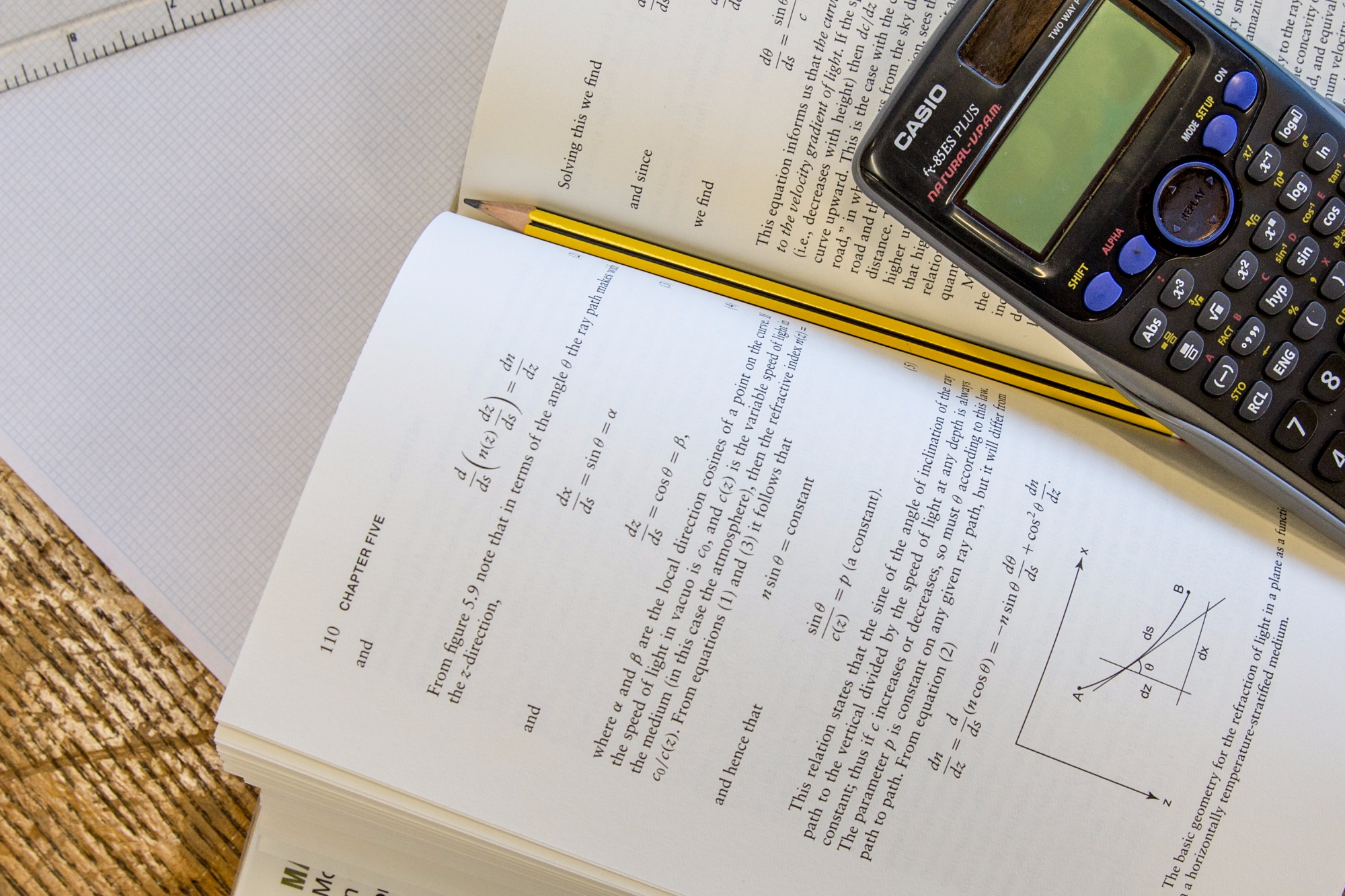• ""

# Mathematics#### Introduction

We encourage our pupils to develop an appreciation and enjoyment of both the pattern and power of Mathematics, and ensure that every pupil is given the opportunity to achieve his/her mathematical potential to the full. The Department believes that to involve the students actively in their learning will help foster independent thinking and informed planning.

Mathematics is creative as well as functional. The Department aims to teach in a meaningful context whilst providing opportunities for the students to use their skills creatively through problem solving and investigation. We endeavour to provide a variety of experience and activities for each topic: observation, talking and listening, reflection, reading and writing all have their place in the Mathematics classroom.

#### Co-Curricular Enrichment

• Maths Challenges (Junior, Intermediate, Senior)
• UK Team Maths Challenge competition
• Maths Kangaroo and Maths Olympiad (follows on from Maths Challenges)
• Puzzle Club
• Maths Forum (discussion around a maths topic or idea)
• Use of ICT facilities within school for research about current maths activity
• Annual trips to:

Woolsthorpe Manor, Sir Isaac Newton’s house
Bletchley Park
Maths Inspiration
GCSE Maths in Action Conference
A-Level Maths in Action Conference

• Lectures and visits organised by the school (e.g. Gresham College Popular Maths Lectures)
• Maths master classes
• Summer school for exceptionally able

#### Form 3 to Upper 4th(Years 7 to 9)

In Years 7 to 9 and throughout the GCSE years, students will develop the ability to describe, tackle and ultimately solve problems that require the application of mathematical knowledge and techniques.  Consequently the main approach to teaching and learning Mathematics will be based on problem solving.

Form 3
Topics include:

Analysing and displaying data; Number skills; Equations, functions and formulae; Fractions; Decimals; Angles and shapes; Equations; Multiplicative reasoning; Sequences and graphs; Perimeter, area and volume

Lower 4th
Topics include:

Factors and powers; Working with powers; 2D shapes and 3D solids; Fractions, decimals and percentages; Probability; Transformations; Real-life graphs; Constructions and loci; Scale drawings and measurements; Graphs

Upper 4th
Topics include:

Powers and roots; Quadratics; Inequalities, equations and formulae; Collecting and analysing data; Multiplicative reasoning; Non-linear graphs; Accuracy and measures; Graphical solutions; Trigonometry; Mathematical reasoning

#### GCSE

GCSE students are assessed in three 1 and a half hour long papers on the following topics:

• Number
• Algebra
• Ratio, proportion and rates of change
• Geometry and measures
• Probability
• Statistics

In addition to the Mathematics course, extra topics are studied throughout years 10 and 11 to provide enrichment and to stretch and challenge the students.

Students are also given the opportunity to take part in the annual UKMT Intermediate Mathematics Challenge event.

#### A Level

In the Sixth Form we encourage pupils to develop further their understanding of Mathematics and mathematical processes in a way that promotes both confidence and enjoyment. A priority will be the teaching of technical skills in algebra and calculus that are needed for real progress in the subject and, in particular, the smooth transition to university courses.

Examples of programmes of study for A-level Maths are given in the table below.

 A Level Mathematics A Level Mathematics Transitus AS Course Modules Core Mathematics  C1             Core Mathematics C1 Core Mathematics  C2             Core Mathematics C2 Mechanics M1                         Probability & Statistics S1 Sixth Form A2 Course Modules Core Mathematics C3 Core Mathematics C4 Probability & Statistics 1 Core Mathematics C3 Core Mathematics C4 Mechanics M1

#### Further Maths

The programme of study for Further Maths includes Core Mathematics, Mechanics, Probability and Statistics, Further Pure Mathematics and Discrete Mathematics.

The programme of study for Further Maths is given in the table below.

 A Level Mathematics & A Level Further Mathematics Transitus AS Course Modules (June Sitting) Core Mathematics C1 Core Mathematics C2 Core Mathematics C3 Core Mathematics C4 Mechanics M1 Probability & Statistics S1 Sixth Form A2 Course Modules (January Sitting) Further Pure Mathematics FP1 Mechanics M2 Probability & Statistics S2 Sixth Form A2 Course Modules (June Sitting) Further Pure Mathematics FP2 Further Pure Mathematics FP3 Mechanics M3 Probability & Statistics S3 Discrete Mathematics D1